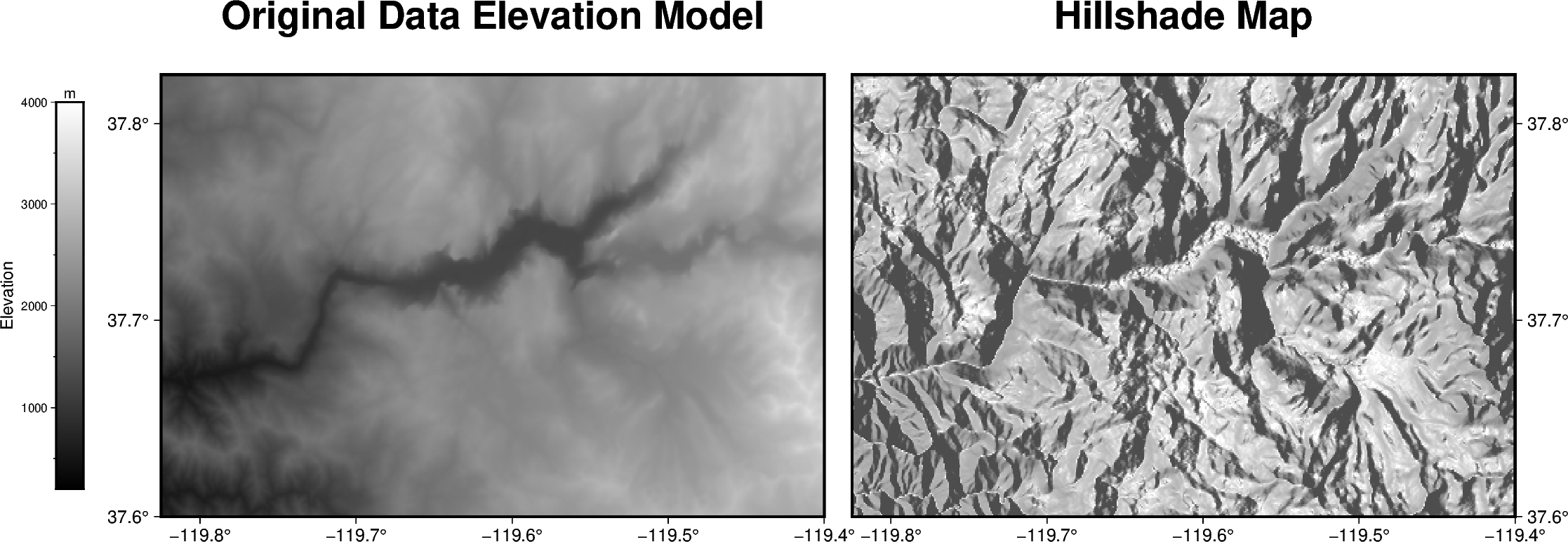The pygmt.grdgradient method calculates the gradient of a grid file. In the example shown below we will see how to calculate a hillshade map based on a Data Elevation Model (DEM). As input pygmt.grdgradient gets a xarray.DataArray object or a path string to a grid file, calculates the respective gradient and returns it as an xarray.DataArray object. We will use the radiance parameter in order to set the illumination source direction and altitude.Out:

<IPython.core.display.Image object>

import pygmt

# Define region of interest around Yosemite valley
region = [-119.825, -119.4, 37.6, 37.825]

# Load sample grid (3 arc second global relief) in target area

# calculate the reflection of a light source projecting from west to east
# (azimuth of 270 degrees) and at a latitude of 30 degrees from the horizon

fig = pygmt.Figure()
# define figure configuration
pygmt.config(FORMAT_GEO_MAP="ddd.x", MAP_FRAME_TYPE="plain")

# --------------- plotting the original Data Elevation Model -----------

pygmt.makecpt(cmap="gray", series=[200, 4000, 10])
fig.grdimage(
grid=grid,
projection="M12c",
frame=['WSrt+t"Original Data Elevation Model"', "xa0.1", "ya0.1"],
cmap=True,
)

fig.colorbar(position="JML+o1.4c/0c+w7c/0.5c", frame=["xa1000f500+lElevation", "y+lm"])

# --------------- plotting the hillshade map -----------

# Shift plot origin of the second map by 12.5 cm in x direction
fig.shift_origin(xshift="12.5c")

pygmt.makecpt(cmap="gray", series=[-1.5, 0.3, 0.01])
fig.grdimage(
grid=dgrid,
projection="M12c",